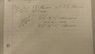# Problem 1.1a from Griffith's E+M

denjay

## Homework Statement

Using the definitions 1.1 and 1.4 show that the dot product and cross product are distributive when

(a) the 3 vectors are coplanar

## Homework Equations

$\vec{A}\cdot\vec{B} = AB\cos(\theta)$
$\vec{A}\times\vec{B} = AB\sin(\theta)\hat{n}$

## The Attempt at a Solution

See attachment.

Not exactly sure of where to go from here, or if I'm even going in the right direction. I do want to do this without using components though as the book never used them when introducing the dot product or vectors.

Haven't attempted cross product yet but I'm assuming it would be using a similar method to the dot product.

#### Attachments

•IMAG0003.jpg
16.4 KB · Views: 437
Last edited:

Careful, in the equation $\mathbf{A} \cdot (\mathbf{B} + \mathbf{C}) = |\mathbf{A}||(\mathbf{B} + \mathbf{C})|\cos\theta$, $\theta$ is the angle between $\mathbf{A}$ and $\mathbf{B} + \mathbf{C}$; but in your diagram, it looks like you are using it to represent the angle between $\mathbf{B}$ and $\mathbf{C}$.
Instead, try letting $\theta$ be the angle between $\mathbf{A}$ and $\mathbf{B} + \mathbf{C}$, $\alpha$ be the angle between $\mathbf{A}$ and $\mathbf{B}$ and $\beta$ be the angle between $\mathbf{A}$ and $\mathbf{C}$, then what you want to prove is that
$$|\mathbf{A}||(\mathbf{B} + \mathbf{C})|\cos\theta = |\mathbf{A}||\mathbf{B}|\cos\alpha + |\mathbf{A}||\mathbf{C}|\cos\beta$$